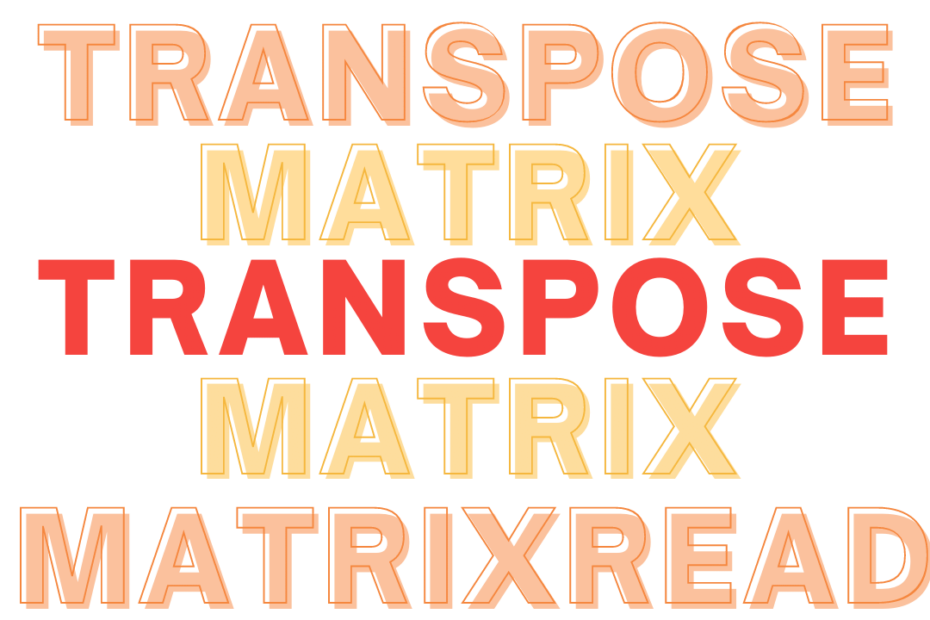# Transpose a Matrix

• DSA

Given a matrix of N dimensions, and we need to transpose it. One of the standard problem on matrices. Don’t confuse Transpose with Rotation, the rotation is normally performed on the X-Y axis while in a transpose, the matrix is flipped on its diagonal.

Example:

## Solution Approach

This is simple swapping but, its a matrix and the swapping takes place over the diagonal

• Simple swapping
• Try not to use extra space [constant sapce]
• Matrix requires minimum of two loops [O(N^2)]

## Code to Transpose a Square Matrix

``````/*Code in C++*/
#include <iostream>
using namespace std;

int main()
{
int i, j, t, N = 4;
int A[N][N] = {{1, 2, 3, 4},
{5, 5, 5, 5},
{6, 7, 8, 9},
{10, 10, 10, 10}};

for (i = 0; i < N; i++)
{
for (j = i + 1; j < N; j++)
{
t = A[i][j];
A[i][j] = A[j][i];
A[j][i] = t;
}
}

for (i = 0; i < N; i++)
{
for (j = 0; j < N; j++)
cout << A[i][j] << " ";
cout << endl;
}

return 0;
}``````

Output

``````1 5 6 10
2 5 7 10
3 5 8 10
4 5 9 10``````

Yes, we did it with Constant Space and only two loops but, this works only for Square matrices where the no.of columns and rows are the same.

## Transposing a Rectangular Matrix

Here are a few points to consider,

• Direct swapping dosen’t work
• We need to use extra space
• Dimensions of input and output matrices are different
• Can do everything within too loops itself.

## Pseudocode

2. Initialize a new Matrix
3. Exchange each number as we iterate `A[i][j] = B[j][i];`

We are actually swapping here and the dimensions of the new matrix are reverse of the input matrix i.e if the input matrix has 3 rows and 4 columns the new/transposed matrix will have 4 rows and 3 columns.

## Code to Transpose a Rectangular Matrix

``````/*Code in C++*/
#include <iostream>
using namespace std;

int main()
{
int M = 3, N = 4, i, j;
int A[M][N] = {{1, 2, 3, 4},
{5, 6, 7, 8},
{9, 10, 11, 12}};
int B[N][M];

for (i = 0; i < N; i++)
for (j = 0; j < M; j++)
B[i][j] = A[j][i];

for (i = 0; i < N; i++)
{
for (j = 0; j < M; j++)
cout << B[i][j] << " ";
cout << endl;
}

return 0;
}``````

## Conclusion

Unlike a square, the rectangle program works for both square and rectangle. Comparing both Square is more optimized as it doesn’t use any extra space.
Happy Coding!